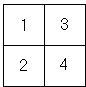# Plane Filling Curves: the Lebesgue Curve

It is not very difficult to show that Hilbert's and Peano's plane filling curves are nowhere differentiable. Judging by their polygonal approximations that turn incessantly, one may well expect the non-differentiability of the limit. However, the visual clues may be deceptive.

The applet below illustrates the generation of the Lebesgue plane filling curve which is differentiable almost everywhere. Who could perceive this by watching?

(To see the progress of polygonal approximations keep clicking in the applet area.)

### If you are reading this, your browser is not set to run Java applets. Try IE11 or Safari and declare the site https://www.cut-the-knot.org as trusted in the Java setup.What if applet does not run?

Geometrically, the approximations join the centers of the subsquares in the order "top-down, left-right":As common, a curve obtained at one step is squeezed into smaller square on the next. The four diminished copies of the curve are joined in the same order of constructions.

Analytically, the curve is related to the Cantor set $C$ and the "stair-case" function.

Recollect that the numbers in the Cantor set admit a ternary expansion, without digit $1$. Every number $c\in C$ can be expressed as

$c = 0.(2t_{1})(2t_{2})(2t_{3})\ldots,$

where $t_{j}, j = 1, 2, \ldots$ are binary digits, either $0$ or $1$.

The Cantor set can be mapped on the unit square $[0,1]\times [0,1]$ surjectively (i.e. "onto") by means of

$0.(2t_{1})(2t_{2})(2t_{3})\ldots \mapsto (0.t_{1}t_{3}\ldots, 0.t_{2}t_{4}\ldots)$

This mapping $f: C \rightarrow [0,1]\times [0,1]$ is obviously, although perhaps surprisingly, surjective because both coordinates of any point $(x,y)$ in the unit square admit a binary expansion; and the two may be interlaced into a single member of $C$ in a reverse of the definition of $f$.

In 1904, H. Lebesgue extended the mapping $f$ by linear interpolation from $C$ to $[0,1]$ in the manner of construction of the Cantor stair-case function. Let $(a,b)$ be one of the intervals removed in the construction of the Cantor set. Then for $t\in (a,b)$ define

$f(t) = \frac{1}{b-a}(f(b)(t-a) + f(a)(b-t)).$

By the construction, $f$, being linear, is differentiable on every such interval $(a,b)$ and, since the measure ("length") of the Cantor set is $0$, it is differentiable "almost everywhere" on the unit interval $[0,1]$.

This is a nice and unexpected result whose veracity is pretty obvious analytically. It may be worth spending some time to make sure that the analytic and geometric aspects of the construction do match each other.

### Reference

1. H. Sagan, Space-Filling Curves, Springer-Verlag, 1994### Plane Filling Curves